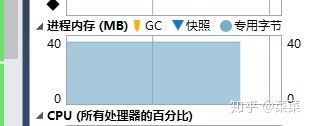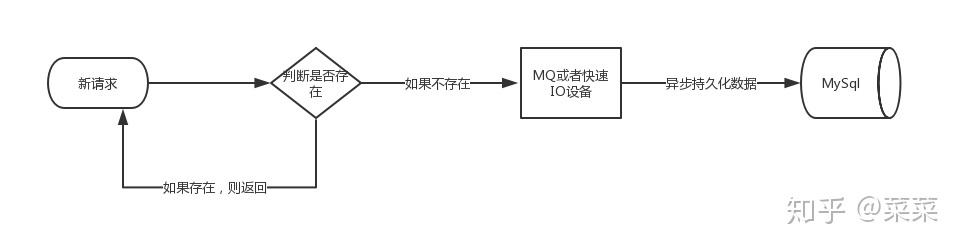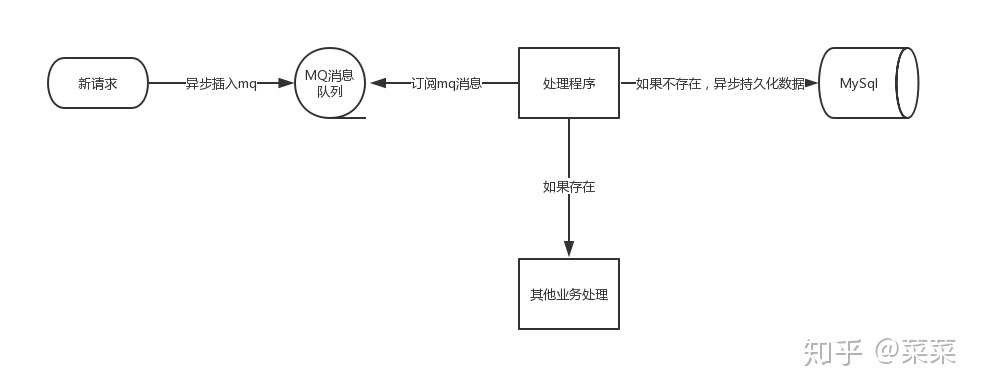# 略微加速

## 略速 - 互联网笔记

2021-03-26 leiting (171阅读)

### 定义

PV是page view的缩写，即页面浏览量，通常是衡量一个网络新闻频道或网站甚至一条网络新闻的主要指标。网页浏览数是评价网站流量最常用的指标之一，简称为PV
UV是unique visitor的简写，是指通过互联网访问、浏览这个网页的自然人。

pv相比较uv来说，技术上比较容易一些，今天咱们就来说一说uv的统计，为什么说uv的统计相对来说比较难呢，因为uv涉及到同一个标准下的自然人的去重，尤其是一个uv千万级别的网站，设计一个好的uv统计系统也许并非想象的那么容易。

### 基于DB方案

```if exists( select 1 from table where ip='ip' and dayid=dayid )
Begin
return 0
End
else
Begin
insert into table .......
End```

### 优化方案

1. 每次请求都需要判断是否已经存在相同的uv记录

2. 持久化uv数据不能影响正常的业务

3. uv数据的准确性可以忍受一定程度的误差

### 优化哈希表

```class BloomFilter
{
BitArray container = null;
public BloomFilter(int length)
{
container = new BitArray(length);
}

public void Set(string key)
{
var h1 = Hash1(key);
var h2 = Hash2(key);
var h3 = Hash3(key);
var h4 = Hash4(key);
container[h1] = true;
container[h2] = true;
container[h3] = true;
container[h4] = true;

}
public bool Get(string key)
{
var h1 = Hash1(key);
var h2 = Hash2(key);
var h3 = Hash3(key);
var h4 = Hash4(key);

return container[h1] && container[h2] && container[h3] && container[h4];
}

//模拟哈希函数1
int Hash1(string key)
{
int hash = 5381;
int i;
int count;
char[] bitarray = key.ToCharArray();
count = bitarray.Length;
while (count > 0)
{
hash += (hash << 5) + (bitarray[bitarray.Length - count]);
count--;
}
return (hash & 0x7FFFFFFF) % container.Length;

}
int Hash2(string key)
{
int seed = 131; // 31 131 1313 13131 131313 etc..
int hash = 0;
int count;
char[] bitarray = (key+"key2").ToCharArray();
count = bitarray.Length;
while (count > 0)
{
hash = hash * seed + (bitarray[bitarray.Length - count]);
count--;
}

return (hash & 0x7FFFFFFF)% container.Length;
}
int Hash3(string key)
{
int hash = 0;
int i;
int count;
char[] bitarray = (key + "keykey3").ToCharArray();
count = bitarray.Length;
for (i = 0; i < count; i++)
{
if ((i & 1) == 0)
{
hash ^= ((hash << 7) ^ (bitarray[i]) ^ (hash >> 3));
}
else
{
hash ^= (~((hash << 11) ^ (bitarray[i]) ^ (hash >> 5)));
}
count--;
}

return (hash & 0x7FFFFFFF) % container.Length;

}
int Hash4(string key)
{
int hash = 5381;
int i;
int count;
char[] bitarray = (key + "keykeyke4").ToCharArray();
count = bitarray.Length;
while (count > 0)
{
hash += (hash << 5) + (bitarray[bitarray.Length - count]);
count--;
}
return (hash & 0x7FFFFFFF) % container.Length;
}
}```

```            BloomFilter bf = new BloomFilter(200000000);
int exsitNumber = 0;
int noExsitNumber = 0;

for (int i=0;i < 10000000; i++)
{
string key = \$"ip_{i}";
var isExsit= bf.Get(key);
if (isExsit)
{
exsitNumber += 1;
}
else
{
bf.Set(key);
noExsitNumber += 1;
}
}
Console.WriteLine(\$"判断存在的数据量：{exsitNumber}");
Console.WriteLine(\$"判断不存在的数据量：{noExsitNumber}");```

```判断存在的数据量：7017### 异步优化